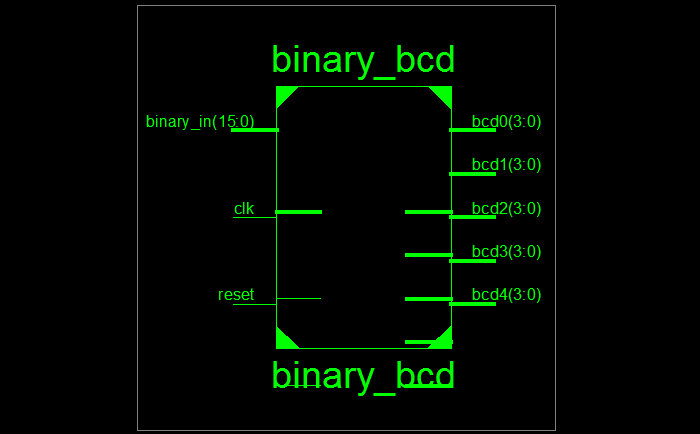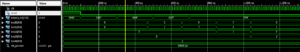# VHDL Code for Binary to BCD Converter

### Binary to BCD Converter

Some times we need to display the output in a seven-segment display. For that purpose we will convert binary to BCD.

To translate from binary to BCD, we can employ the shift-and-add-3 algorithm:

Left-shift the (n-bit) binary number one bit.
If n shifts have taken place, the number has been fully expanded, so exit the algorithm.
If the binary value of any of the BCD columns is greater than or equal to 5, add 3.

### VHDL Code for Binary to BCD Converter

```library ieee;
use ieee.std_logic_1164.all;
use ieee.std_logic_unsigned.all;

entity binary_bcd is
generic(N: positive := 16);
port(
clk, reset: in std_logic;
binary_in: in std_logic_vector(N-1 downto 0);
bcd0, bcd1, bcd2, bcd3, bcd4: out std_logic_vector(3 downto 0)
);
end binary_bcd ;

architecture behaviour of binary_bcd is
type states is (start, shift, done);
signal state, state_next: states;

signal binary, binary_next: std_logic_vector(N-1 downto 0);
signal bcds, bcds_reg, bcds_next: std_logic_vector(19 downto 0);
-- output register keep output constant during conversion
signal bcds_out_reg, bcds_out_reg_next: std_logic_vector(19 downto 0);
-- need to keep track of shifts
signal shift_counter, shift_counter_next: natural range 0 to N;
begin

process(clk, reset)
begin
if reset = '1' then
binary <= (others => '0');
bcds <= (others => '0');
state <= start;
bcds_out_reg <= (others => '0');
shift_counter <= 0;
elsif falling_edge(clk) then
binary <= binary_next;
bcds <= bcds_next;
state <= state_next;
bcds_out_reg <= bcds_out_reg_next;
shift_counter <= shift_counter_next;
end if;
end process;

convert:
process(state, binary, binary_in, bcds, bcds_reg, shift_counter)
begin
state_next <= state;
bcds_next <= bcds;
binary_next <= binary;
shift_counter_next <= shift_counter;

case state is
when start =>
state_next <= shift;
binary_next <= binary_in;
bcds_next <= (others => '0');
shift_counter_next <= 0;
when shift =>
if shift_counter = N then
state_next <= done;
else
binary_next <= binary(N-2 downto 0) & 'L';
bcds_next <= bcds_reg(18 downto 0) & binary(N-1);
shift_counter_next <= shift_counter + 1;
end if;
when done =>
state_next <= start;
end case;
end process;

bcds_reg(19 downto 16) <= bcds(19 downto 16) + 3 when bcds(19 downto 16) > 4 else
bcds(19 downto 16);
bcds_reg(15 downto 12) <= bcds(15 downto 12) + 3 when bcds(15 downto 12) > 4 else
bcds(15 downto 12);
bcds_reg(11 downto 8) <= bcds(11 downto 8) + 3 when bcds(11 downto 8) > 4 else
bcds(11 downto 8);
bcds_reg(7 downto 4) <= bcds(7 downto 4) + 3 when bcds(7 downto 4) > 4 else
bcds(7 downto 4);
bcds_reg(3 downto 0) <= bcds(3 downto 0) + 3 when bcds(3 downto 0) > 4 else
bcds(3 downto 0);

bcds_out_reg_next <= bcds when state = done else
bcds_out_reg;

bcd4 <= bcds_out_reg(19 downto 16);
bcd3 <= bcds_out_reg(15 downto 12);
bcd2 <= bcds_out_reg(11 downto 8);
bcd1 <= bcds_out_reg(7 downto 4);
bcd0 <= bcds_out_reg(3 downto 0);

end behaviour;
```

### VHDL Teshbench Code for Binary to BCD Converter

```LIBRARY ieee;
USE ieee.std_logic_1164.ALL;

ENTITY tb_bcd IS
END tb_bcd;

ARCHITECTURE behavior OF tb_bcd IS

-- Component Declaration for the Unit Under Test (UUT)

COMPONENT binary_bcd
PORT(
clk : IN  std_logic;
reset : IN  std_logic;
binary_in : IN  std_logic_vector(15 downto 0);
bcd0 : OUT  std_logic_vector(3 downto 0);
bcd1 : OUT  std_logic_vector(3 downto 0);
bcd2 : OUT  std_logic_vector(3 downto 0);
bcd3 : OUT  std_logic_vector(3 downto 0);
bcd4 : OUT  std_logic_vector(3 downto 0)
);
END COMPONENT;

--Inputs
signal clk : std_logic := '0';
signal reset : std_logic := '0';
signal binary_in : std_logic_vector(15 downto 0) := (others => '0');

--Outputs
signal bcd0 : std_logic_vector(3 downto 0);
signal bcd1 : std_logic_vector(3 downto 0);
signal bcd2 : std_logic_vector(3 downto 0);
signal bcd3 : std_logic_vector(3 downto 0);
signal bcd4 : std_logic_vector(3 downto 0);

-- Clock period definitions
constant clk_period : time := 10 ns;

BEGIN

-- Instantiate the Unit Under Test (UUT)
uut: binary_bcd PORT MAP (
clk => clk,
reset => reset,
binary_in => binary_in,
bcd0 => bcd0,
bcd1 => bcd1,
bcd2 => bcd2,
bcd3 => bcd3,
bcd4 => bcd4
);

-- Clock process definitions
clk_process :process
begin
clk <= '0';
wait for clk_period/2;
clk <= '1';
wait for clk_period/2;
end process;

-- Stimulus process
stim_proc: process
begin
-- hold reset state for 100 ns.
reset <= '1';
wait for 100 ns;
reset <= '0';

binary_in <= "0000000000001111";
wait for 200 ns;

binary_in <= "0000000001001111";
wait for 200 ns;

binary_in <= "0000000001111111";
wait for 200 ns;

binary_in <= "0000111101001111";
wait for 2000 ns;

end process;

END;

```

### Simulation Waveform Result for Binary to BCD Converter### 3 thoughts on “VHDL Code for Binary to BCD Converter”

1.I am using an Altera MAX10M25 CLPD / FPGA. On modelsim it works fine without Errors. I am going to expand the converter to 32 bits. I know, it is a little bit more too to as just changing N.
My next step would be to expand the BCD Code to 8 bit ASCII numbers. Which should be a realy simple Task.

Thank You for these nice converter.

2.Very nice!
How can I change the code for support in 17 bit binary input?
i changed N to 16 (16:0) but it still limit the output by 65535.

Thanks
Chagai

•